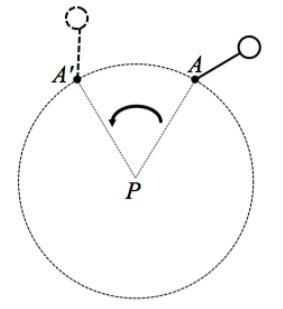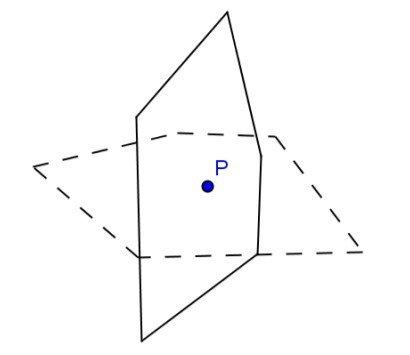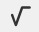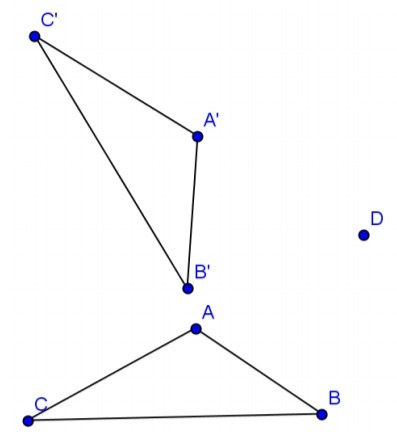PSA2PDW - Problem Sets---Geometry, M1 Lesson 12 (G.CO.A.2)

An example of a rotation applied to a figure and its image are provided. Use this representation to answer the questions that follow. For each question, a pair of figures (pre-image and image) is given as well as the center of rotation. For each question, identify and draw the following:

1. The circle that determines the rotation, using any point on the pre-image and its image.
2. An angle, created with three points of your choice, which demonstrates the angle of rotation.

 Example of a Rotation: Pre-image: (solid line) image: (dotted line) Center of rotation: P Angle of rotation:Pre-image: (solid line) Image: (dotted line) Center of rotation: PAngle of rotation: _______

Write your answer using the "WIRIS editor" buttonModified from EngageNY ©Great Minds Disclaimer

 Pre-image:Image:Center: DAngle of rotation: ____________

Write your answer using the "WIRIS editor" buttonModified from EngageNY ©Great Minds Disclaimer